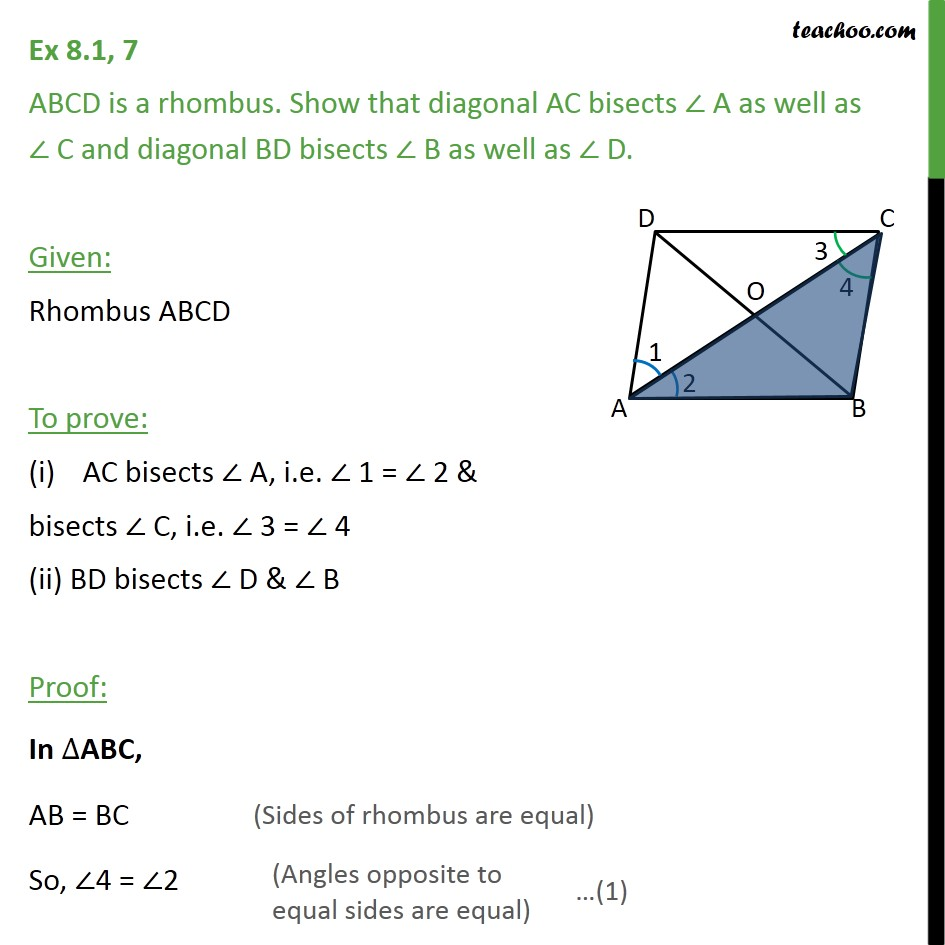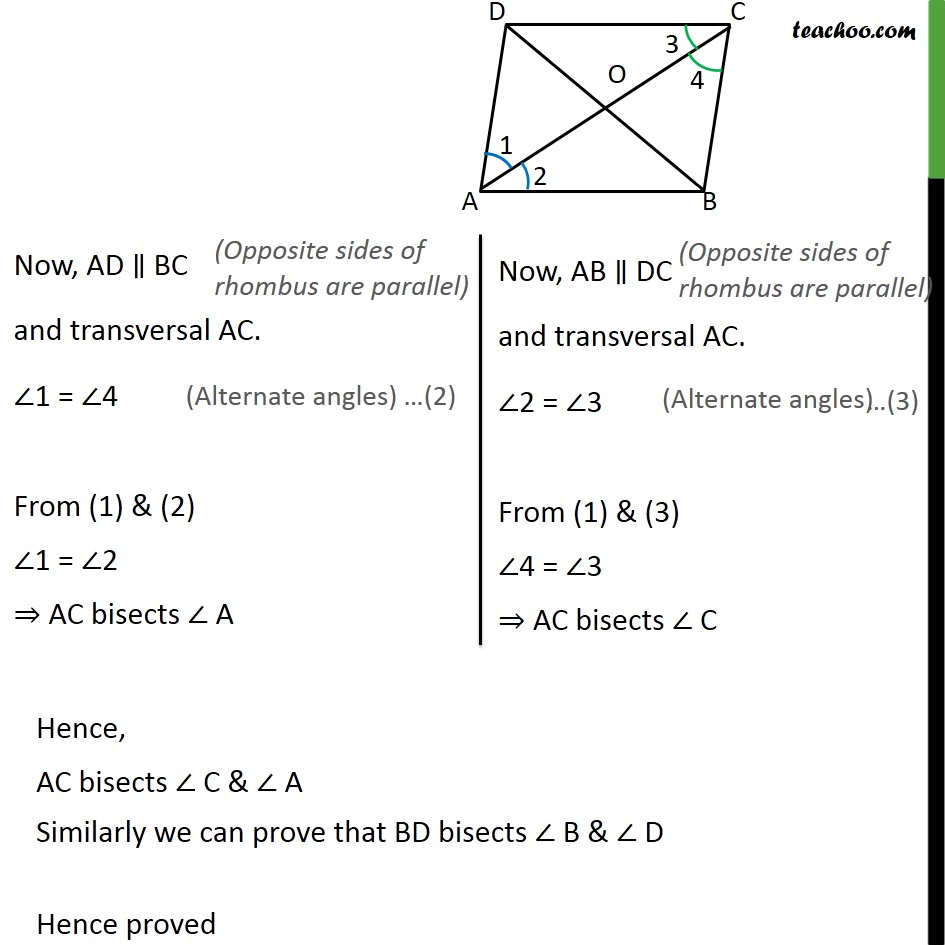1. Chapter 8 Class 9 Quadrilaterals (Term 2)
2. Serial order wise
3. Ex 8.1

Transcript

Ex 8.1, 7 ABCD is a rhombus. Show that diagonal AC bisects ∠ A as well as ∠ C and diagonal BD bisects ∠ B as well as ∠ D. Given: Rhombus ABCD To prove: AC bisects ∠ A, i.e. ∠ 1 = ∠ 2 & bisects ∠ C, i.e. ∠ 3 = ∠ 4 (ii) BD bisects ∠ D & ∠ B Proof: In ∆ABC, AB = BC So, ∠4 = ∠2 Hence, AC bisects ∠ C & ∠ A Similarly we can prove that BD bisects ∠ B & ∠ D Hence proved